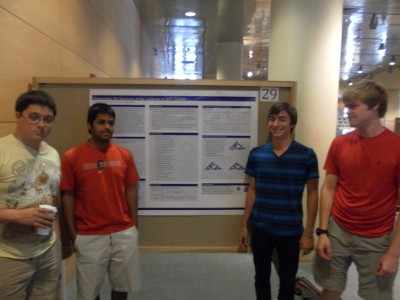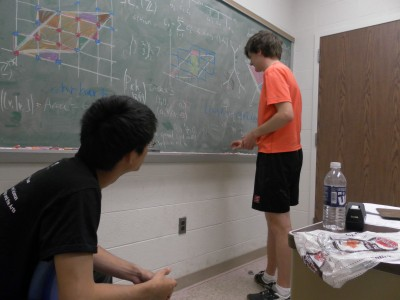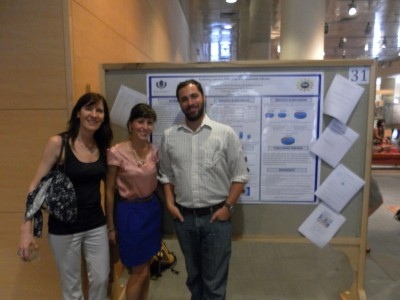# Gaps in the Spectrum of the Laplacian on 3N-Gaskets

arXiv.org

## Project

Project Information

# Determining the Spectrum of the Laplacian on 3N-Gaskets## Overview

The Laplacian is the central object of analysis on fractals. While most work on the Laplacian has been focused on computing the Laplacian spectrum on specific fractals, this group instead found the spectrum on an entire class of fractals—the 3N-Gaskets. This is the class of finitely ramified fractal 3N-Gons that are the attractors of iterated function systems containing 3N contraction mappings. For example, the 3-Gasket is the Sierpinski triangle.

The Laplacian on the fractal, and thus its eigenvalues, must be studied by examining the graph Laplacian on approximating graphs. The group found a function R(z) relating the Laplacian eigenvalues on consecutive graph approximations. They used R(z) along with the eigenvalues of the Laplacian on graph approximations to derive part of the spectrum on the next level. The rest of the spectrum was found using an “exceptional set”— a set of values which the group provided for arbitrary N. By repeating this infinitely many times, the spectrum of the Laplacian on the fractal could be found.

arXiv.org

# Frobenius Splitting of Projective Toric Varieties## Overview

A toric variety is an algebraic variety containing the algebraic torus (C*)n as an open dense subset such that the action of the torus extends to the whole variety. Every n-dimensional toric variety can be associated to a fan, which can be given as a set of primitive vectors in an n-dimensional lattice N. Because of this association, many properties of toric varieties can be studied using combinatorial methods. This group was interested in determining which projective toric varieties are Frobenius split. If a projective toric variety is Frobenius split, it has many nice properties. For example, it can be given as the solution set to homogeneous degree two polynomials.

Sam Payne proved in 2008 that a toric variety is Frobenius split if and only if an associated polytope called the splitting polytope contains representatives of every residue class of (1/q)M/M where M is the dual lattice to N. The group’s goal was to use this characterization of Frobenius splitting to classify the Frobenius split projective toric varieties in n dimensions. Given a trivalent tree where edges are labeled with variables and integers, it’s possible to construct the fan of a toric variety. They determined which toric varieties arising in this way are Frobenius split for certain classes of edge labelings.

# Lyapunov Exponents of Multiplicative Stochastic Processes## Overview

This group considered the stability of matrix Lie group valued stochastic differential equations, dXt = AXtdt + BXtdt. Random dynamical systems such as this arise in many applications (e.g., oceanic turbulence, helicopter blade motion, light in random channels, wireless networks) in which stability is of practical and theoretical concern. The stability of the zero solution, Xt = 0, is determined by the top Lyapunov exponent. But in practice, analytic calculations of the Lyapunov exponent are often impossible, so time discrete approximations and simulations are necessary. Oceledet’s famous multiplicative ergodic theorem ensures that the Lyapunov exponent of Xt is almost surely constant. But the Lyapunov exponent of a time discrete approximation is itself a random variable. The mean of this random variable has been studied. The group provided estimates on the variance, distribution and rate of convergence in certain numerical approximation methods. In addition, they expanded on and provided simulations for recent results regarding the top Lyapunov exponent of certain Lie group valued SDEs.

# Exploring Learning Difficulties in Multivariable Calculus## Contributors

Cathy Matta, Gabriel Feinberg, and Fabiana Cardetti

## Overview

This pilot study used student perceptions about their understanding of mathematics to guide the development of learning aids for multivariable calculus classes. Studies on the use of computer technology in advanced mathematics classrooms have shown that technology can help with the understanding of abstract concepts (Godaszi, Elahe Aminifar, & Bakhshalizadeh, 2009; Verner, Aroshas, & Berman, 2008). In addition, other researchers have found that using real-world applications and Inquiry Based Learning (IBL) projects can also help students not only with their learning but also with their enjoyment of mathematics (Hassi & Laursen, 2009; Spronken-Smith, Walker, Batchelor, O’Steen, & Angelo, 2012; Stillman, Galbraith, Brown, Edwards, 2007). In this study, these approaches were used in conjunction with students’ perceptions (Pierce, Stacey, & Barkatsas, 2007; Schoenfeld, 1989; Szydlik, 2000) to develop learning aids for multivariable calculus.

## Presentation

Exploring Learning Difficulties in Multivariable Calculus